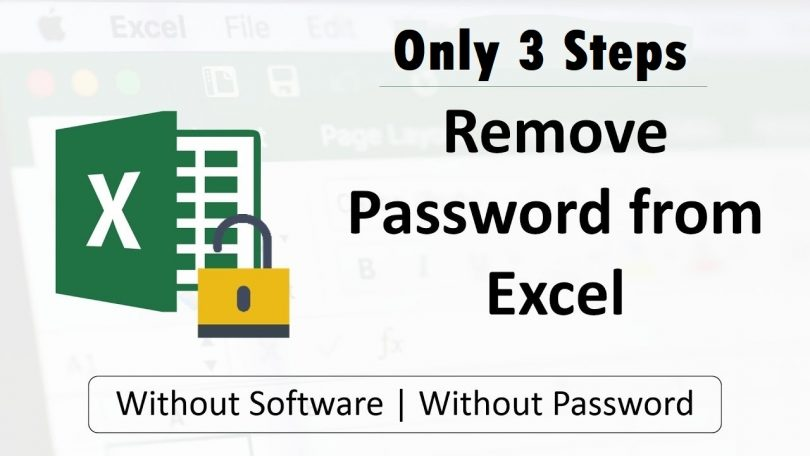Trendy

# How to Unlock Excel File Without PasswordWritten by

Note :

This Unlock Trick work in Microsoft Excel 2010 and Earlier Versions but in MS Excel 2013 Not Working.

Don’t Worry For the Password Protected Excel Sheet

Like You have a Password Protected Excel File you are unable to Edit Data in Excel Worksheet. If you have not remember the Password or don’t know the Password of Excel Sheet that time this step very much helpful to Edit Data in Excel File.

You have to Follow the Simple Steps to Unlock the Excel Sheet.

Step 1 :

First Press the Alt + F11

Then One New Dialog Tab Sheet Opened. Like that

Step 2 :

In the above picture VBA Project Click on This Work Book then appearing the Codding Part.

after that Coding part you have to Paste Below Code. Do not Change. Just Only Copy Paste.

Dim i As Integer, j As Integer, k As Integer
Dim l As Integer, m As Integer, n As Integer
Dim i1 As Integer, i2 As Integer, i3 As Integer
Dim i4 As Integer, i5 As Integer, i6 As Integer
On Error Resume Next
For i = 65 To 66: For j = 65 To 66: For k = 65 To 66
For l = 65 To 66: For m = 65 To 66: For i1 = 65 To 66
For i2 = 65 To 66: For i3 = 65 To 66: For i4 = 65 To 66
For i5 = 65 To 66: For i6 = 65 To 66: For n = 32 To 126
ActiveSheet.Unprotect Chr(i) & Chr(j) & Chr(k) & _
Chr(l) & Chr(m) & Chr(i1) & Chr(i2) & Chr(i3) & _
Chr(i4) & Chr(i5) & Chr(i6) & Chr(n)
If ActiveSheet.ProtectContents = False Then
MsgBox “One usable password is ” & Chr(i) & Chr(j) & _
Chr(k) & Chr(l) & Chr(m) & Chr(i1) & Chr(i2) & _
Chr(i3) & Chr(i4) & Chr(i5) & Chr(i6) & Chr(n)
Exit Sub
End If
Next: Next: Next: Next: Next: Next
Next: Next: Next: Next: Next: Next
End Sub

Step 3 :

Click on Run Button

And there you go the sheet is unprotected for you now. Also you would be getting a message in the pop up window.

This Message is contains the password which can be used to unprotected the other sheets in the same workbook.

Hope you Enjoy the Trick..